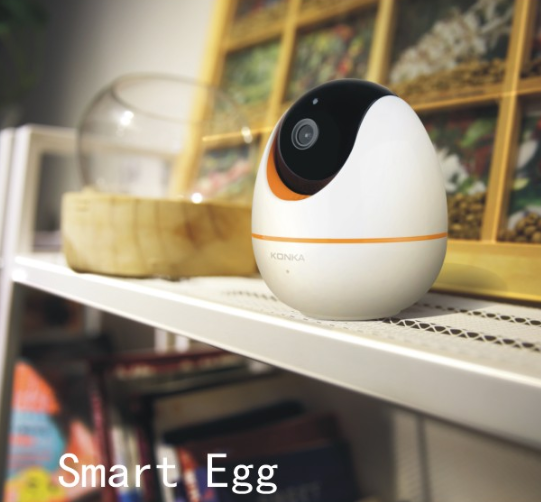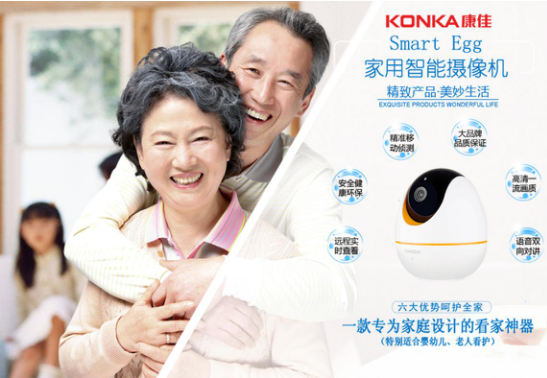|

# 康佳家用摄像机启动城市合伙人招募,奉上首份大礼!

得益于家庭收入日益增加，智能家居在中国市场迅速渗透。根据报告，到2020年，全球智能家居的整体规模将由目前的100亿美元增长至500亿美元，并有望在2030年增至4000亿美元。到2030年，亚洲市场销售额将超过1150亿美元，占据全球市场25%以上的份额。其中，在美国智能监控摄像头Dropcam被Google收购后，家庭安防智能摄像机成智能家居热门品类，市场炙手可热。

战略开局

2016年12月，康佳信息宣布推出KONKA首款家用智能摄像机Smart Egg，正式进军智能家庭安防领域及智能家居领域。康佳信息全称深圳康佳信息网络有限公司，是康佳集团与战略投资者成立的合资公司，旨在通过混合所有制改革、创新激励方式，为康佳品牌的多元化发展探索可持续发展道路。

2017年战略开局之年，阳春三月，康佳信息正式启动全国代理商及城市合伙人招募!并将以全新的身份和商业布局给代理商和消费者展示开拓创新，追求品质，战略发展的活力形象。

招商原则

产品定位：康佳信息智能家居坚持走品质型消费路线。首款推出的家用智能摄像机新品，是基于对中国家庭消费者的喜好分析与研究，智能家庭安防市场发展现状及对未来家庭安防及智能家居的消费趋势而发布的，康佳智能家居致力于为千万家庭用户奉献出性价比最高的产品。

招商原则：寻求全国区域城市的事业合伙人，而不仅仅是定位于家庭安防传统代理商，即以康佳信息作为平台，优选理念一致、有实力、有事业心的核心合作伙伴，通过共同努力，逐渐将康佳智能家居打造成一个以用户为中心的生态型平台。

爆款首推：康佳Smart Egg家用智能摄像机

康佳Smart Egg家用智能摄像机是一款专为家庭设计的看家神器，主要针对家庭安防及智能家居市场，其以创意的“蛋”型设计，致力于为家庭提供安全绿色健康的全心防护，特别适合婴幼儿、老人看护，同时也能广泛应用于各种类型的住宅区(别墅、小区等)、幼儿园、室内场所(店铺、超市、专卖店、营业厅、公司办公室等)等室内监控场所。特色优势：

整体设计：蛋型设计，重心在下，稳固牢靠，一只手刚好拿起。

材质选取：电子材料完全无铅无汞，外壳采用安全无毒的ABS塑料。

色彩选取：光泽的象牙白配上靓丽的橘红色，保持好心情。

镜头选取：全金属3.6mm，200万像素，F2.7光圈。

马达选取：1：64转速，康佳专门定制的小型化电机。

网口选取：采用Mini USB型网口，防止婴幼儿和老人手指受伤。

APP界面：一键式安装，增加二维码，家人无需再配对，直接分享。

神秘功能：对码功能，康佳智能产品可以通过对码功能互联。加盟支持

全国统一零售价格;整体的形象宣传;第一手的市场信息和预测;完善的售前和售后服务;给加盟商各项切实的服务及指导(包括产品的专业知识，市场的前景和销售方法)。

1、强有力的风险保障：康佳信息独特的加盟体系，零风险退换货制度，使加盟代理商无后顾之忧。

2、丰厚的利润保障：凭借康佳知名品牌、高质量的产品、快速完善的服务、合理实在的价格，建立以代理商/城市合伙人为中心的双赢模式，作为加盟代理商获取丰厚利润的保障。

3、严格的市场保护：区域性保护。

4、完善的售后服务：康佳信息以质量为生存，以服务为发展，代理商享有“零”售后，售后无忧。

5、强有力的广告服务：康佳信息网络为代理商提供系统的广告、营销宣传支持，立体式全方位打造品牌产品的影响力。

首份见面大礼!送!送!送!

2017年3月23日-25日，康佳信息网络将隆重亮相亚洲最大影响力广电行业展会之一——2017北京CCBN大展，康佳Smart Egg家用智能摄像机也将在此次展会上惊艳亮相。康佳信息网络在这次展会现场准备了首份见面大礼，加盟预定康佳家用智能摄像机享有超级大礼!(劲爆活动，现场揭晓!)总之一句：只要你敢订，康佳就敢送!数量有限，先订先得!现场签约，还有更多惊喜!(康佳信息网络展台：8A102)

粉丝追随偶像是始于颜值，陷于才华，忠于人品;而经销商选择与企业合作是始于契机，陷于产品，忠于信任。现如今，康佳信息愿与你携手共创智能家居、智能家庭安防锦绣江山!

想要加盟康佳?

如果你在找智能家居好产品，请选择康佳家用智能摄像机!

如果你想找个好品牌，康佳信息支持你!

如果你想拥有好利润，康佳信息满足你!

相信面对市场爆发的2017年，康佳信息通过与代理商的协同作战，必将共同创造出一幅“双赢”的画卷!

`声明：本文由入驻焦点开放平台的作者撰写，除焦点官方账号外，观点仅代表作者本人，不代表焦点立场错误信息举报电话： 400-099-0099，邮箱：jubao@vip.sohu.com，或点此进行意见反馈，或点此进行举报投诉。`A B C D E F G H J K L M N P Q R S T W X Y Z
A - B - C - D - E
• A
• 鞍山
• 安庆
• 安阳
• 安顺
• 安康
• 澳门
• B
• 北京
• 保定
• 包头
• 巴彦淖尔
• 本溪
• 蚌埠
• 亳州
• 滨州
• 北海
• 百色
• 巴中
• 毕节
• 保山
• 宝鸡
• 白银
• 巴州
• C
• 承德
• 沧州
• 长治
• 赤峰
• 朝阳
• 长春
• 常州
• 滁州
• 池州
• 长沙
• 常德
• 郴州
• 潮州
• 崇左
• 重庆
• 成都
• 楚雄
• 昌都
• 慈溪
• 常熟
• D
• 大同
• 大连
• 丹东
• 大庆
• 东营
• 德州
• 东莞
• 德阳
• 达州
• 大理
• 德宏
• 定西
• 儋州
• 东平
• E
• 鄂尔多斯
• 鄂州
• 恩施
F - G - H - I - J
• F
• 抚顺
• 阜新
• 阜阳
• 福州
• 抚州
• 佛山
• 防城港
• G
• 赣州
• 广州
• 桂林
• 贵港
• 广元
• 广安
• 贵阳
• 固原
• H
• 邯郸
• 衡水
• 呼和浩特
• 呼伦贝尔
• 葫芦岛
• 哈尔滨
• 黑河
• 淮安
• 杭州
• 湖州
• 合肥
• 淮南
• 淮北
• 黄山
• 菏泽
• 鹤壁
• 黄石
• 黄冈
• 衡阳
• 怀化
• 惠州
• 河源
• 贺州
• 河池
• 海口
• 红河
• 汉中
• 海东
• 怀来
• I
• J
• 晋中
• 锦州
• 吉林
• 鸡西
• 佳木斯
• 嘉兴
• 金华
• 景德镇
• 九江
• 吉安
• 济南
• 济宁
• 焦作
• 荆门
• 荆州
• 江门
• 揭阳
• 金昌
• 酒泉
• 嘉峪关
K - L - M - N - P
• K
• 开封
• 昆明
• 昆山
• L
• 廊坊
• 临汾
• 辽阳
• 连云港
• 丽水
• 六安
• 龙岩
• 莱芜
• 临沂
• 聊城
• 洛阳
• 漯河
• 娄底
• 柳州
• 来宾
• 泸州
• 乐山
• 六盘水
• 丽江
• 临沧
• 拉萨
• 林芝
• 兰州
• 陇南
• M
• 牡丹江
• 马鞍山
• 茂名
• 梅州
• 绵阳
• 眉山
• N
• 南京
• 南通
• 宁波
• 南平
• 宁德
• 南昌
• 南阳
• 南宁
• 内江
• 南充
• P
• 盘锦
• 莆田
• 平顶山
• 濮阳
• 攀枝花
• 普洱
• 平凉
Q - R - S - T - W
• Q
• 秦皇岛
• 齐齐哈尔
• 衢州
• 泉州
• 青岛
• 清远
• 钦州
• 黔南
• 曲靖
• 庆阳
• R
• 日照
• 日喀则
• S
• 石家庄
• 沈阳
• 双鸭山
• 绥化
• 上海
• 苏州
• 宿迁
• 绍兴
• 宿州
• 三明
• 上饶
• 三门峡
• 商丘
• 十堰
• 随州
• 邵阳
• 韶关
• 深圳
• 汕头
• 汕尾
• 三亚
• 三沙
• 遂宁
• 山南
• 商洛
• 石嘴山
• T
• 天津
• 唐山
• 太原
• 通辽
• 铁岭
• 泰州
• 台州
• 铜陵
• 泰安
• 铜仁
• 铜川
• 天水
• 天门
• W
• 乌海
• 乌兰察布
• 无锡
• 温州
• 芜湖
• 潍坊
• 威海
• 武汉
• 梧州
• 渭南
• 武威
• 吴忠
• 乌鲁木齐
X - Y - Z
• X
• 邢台
• 徐州
• 宣城
• 厦门
• 新乡
• 许昌
• 信阳
• 襄阳
• 孝感
• 咸宁
• 湘潭
• 湘西
• 西双版纳
• 西安
• 咸阳
• 西宁
• 仙桃
• 西昌
• Y
• 运城
• 营口
• 盐城
• 扬州
• 鹰潭
• 宜春
• 烟台
• 宜昌
• 岳阳
• 益阳
• 永州
• 阳江
• 云浮
• 玉林
• 宜宾
• 雅安
• 玉溪
• 延安
• 榆林
• 银川
• Z
• 张家口
• 镇江
• 舟山
• 漳州
• 淄博
• 枣庄
• 郑州
• 周口
• 驻马店
• 株洲
• 张家界
• 珠海
• 湛江
• 肇庆
• 中山
• 自贡
• 资阳
• 遵义
• 昭通
• 张掖
• 中卫

1室1厅1厨1卫1阳台

1
2
3
4
5

0
1
2

1

1

0
1
2
3报名成功，资料已提交审核A B C D E F G H J K L M N P Q R S T W X Y Z
A - B - C - D - E
• A
• 鞍山
• 安庆
• 安阳
• 安顺
• 安康
• 澳门
• B
• 北京
• 保定
• 包头
• 巴彦淖尔
• 本溪
• 蚌埠
• 亳州
• 滨州
• 北海
• 百色
• 巴中
• 毕节
• 保山
• 宝鸡
• 白银
• 巴州
• C
• 承德
• 沧州
• 长治
• 赤峰
• 朝阳
• 长春
• 常州
• 滁州
• 池州
• 长沙
• 常德
• 郴州
• 潮州
• 崇左
• 重庆
• 成都
• 楚雄
• 昌都
• 慈溪
• 常熟
• D
• 大同
• 大连
• 丹东
• 大庆
• 东营
• 德州
• 东莞
• 德阳
• 达州
• 大理
• 德宏
• 定西
• 儋州
• 东平
• E
• 鄂尔多斯
• 鄂州
• 恩施
F - G - H - I - J
• F
• 抚顺
• 阜新
• 阜阳
• 福州
• 抚州
• 佛山
• 防城港
• G
• 赣州
• 广州
• 桂林
• 贵港
• 广元
• 广安
• 贵阳
• 固原
• H
• 邯郸
• 衡水
• 呼和浩特
• 呼伦贝尔
• 葫芦岛
• 哈尔滨
• 黑河
• 淮安
• 杭州
• 湖州
• 合肥
• 淮南
• 淮北
• 黄山
• 菏泽
• 鹤壁
• 黄石
• 黄冈
• 衡阳
• 怀化
• 惠州
• 河源
• 贺州
• 河池
• 海口
• 红河
• 汉中
• 海东
• 怀来
• I
• J
• 晋中
• 锦州
• 吉林
• 鸡西
• 佳木斯
• 嘉兴
• 金华
• 景德镇
• 九江
• 吉安
• 济南
• 济宁
• 焦作
• 荆门
• 荆州
• 江门
• 揭阳
• 金昌
• 酒泉
• 嘉峪关
K - L - M - N - P
• K
• 开封
• 昆明
• 昆山
• L
• 廊坊
• 临汾
• 辽阳
• 连云港
• 丽水
• 六安
• 龙岩
• 莱芜
• 临沂
• 聊城
• 洛阳
• 漯河
• 娄底
• 柳州
• 来宾
• 泸州
• 乐山
• 六盘水
• 丽江
• 临沧
• 拉萨
• 林芝
• 兰州
• 陇南
• M
• 牡丹江
• 马鞍山
• 茂名
• 梅州
• 绵阳
• 眉山
• N
• 南京
• 南通
• 宁波
• 南平
• 宁德
• 南昌
• 南阳
• 南宁
• 内江
• 南充
• P
• 盘锦
• 莆田
• 平顶山
• 濮阳
• 攀枝花
• 普洱
• 平凉
Q - R - S - T - W
• Q
• 秦皇岛
• 齐齐哈尔
• 衢州
• 泉州
• 青岛
• 清远
• 钦州
• 黔南
• 曲靖
• 庆阳
• R
• 日照
• 日喀则
• S
• 石家庄
• 沈阳
• 双鸭山
• 绥化
• 上海
• 苏州
• 宿迁
• 绍兴
• 宿州
• 三明
• 上饶
• 三门峡
• 商丘
• 十堰
• 随州
• 邵阳
• 韶关
• 深圳
• 汕头
• 汕尾
• 三亚
• 三沙
• 遂宁
• 山南
• 商洛
• 石嘴山
• T
• 天津
• 唐山
• 太原
• 通辽
• 铁岭
• 泰州
• 台州
• 铜陵
• 泰安
• 铜仁
• 铜川
• 天水
• 天门
• W
• 乌海
• 乌兰察布
• 无锡
• 温州
• 芜湖
• 潍坊
• 威海
• 武汉
• 梧州
• 渭南
• 武威
• 吴忠
• 乌鲁木齐
X - Y - Z
• X
• 邢台
• 徐州
• 宣城
• 厦门
• 新乡
• 许昌
• 信阳
• 襄阳
• 孝感
• 咸宁
• 湘潭
• 湘西
• 西双版纳
• 西安
• 咸阳
• 西宁
• 仙桃
• 西昌
• Y
• 运城
• 营口
• 盐城
• 扬州
• 鹰潭
• 宜春
• 烟台
• 宜昌
• 岳阳
• 益阳
• 永州
• 阳江
• 云浮
• 玉林
• 宜宾
• 雅安
• 玉溪
• 延安
• 榆林
• 银川
• Z
• 张家口
• 镇江
• 舟山
• 漳州
• 淄博
• 枣庄
• 郑州
• 周口
• 驻马店
• 株洲
• 张家界
• 珠海
• 湛江
• 肇庆
• 中山
• 自贡
• 资阳
• 遵义
• 昭通
• 张掖
• 中卫• 手机• 分享
• 设计
免费设计
• 计算器
装修计算器
• 入驻
合作入驻
• 联系
联系我们
• 置顶
返回顶部